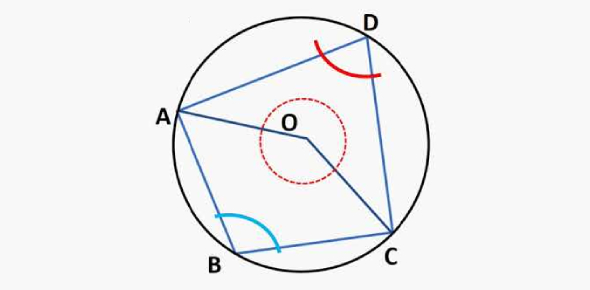8 Questions | Total Attempts: 49Settings• 1.
How many degrees are in a triangle?
• A.

90

• B.

360

• C.

180

• 2.
Which one are a Cyclic Quadrilaterals?
• A.

Option 1

• B.

Option 2

• C.

Option 3

• D.

Option 4

• 3.
What is the sum of the opposite interior angles?
• A.

90

• B.

180

• C.

270

• D.

360

• 4.
Which one is the right answer?
• A.

P=36 q=60

• B.

P=60 q=45

• C.

P=45 q=60

• D.

P=36 q=45

• 5.
What is the value of b and c?
• A.

B=95 c=100

• B.

B=100 c=90

• C.

B=90 c=110

• D.

B=110 c=95

• 6.
Find the value of x,y, and z.
• A.

X=70 y=80 z=95

• B.

X=80 y=73 z=90

• C.

X=73 y=85 z=93

• D.

x=73 y=85 z=95

• 7.
The diagram below shows a cyclic quadrilateral /PQRS and a straight line RST. Calculate the value of /PST.
• A.

150

• B.

120

• C.

50

• D.

30

• 8.
Is the answer below true or false?
• A.

True

• B.

False

Related TopicsBack to top